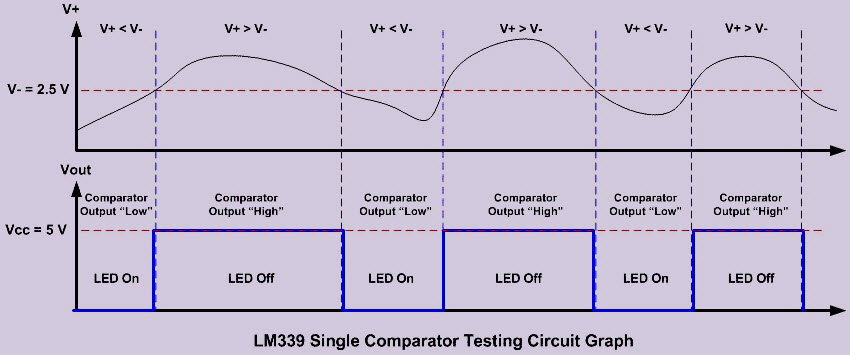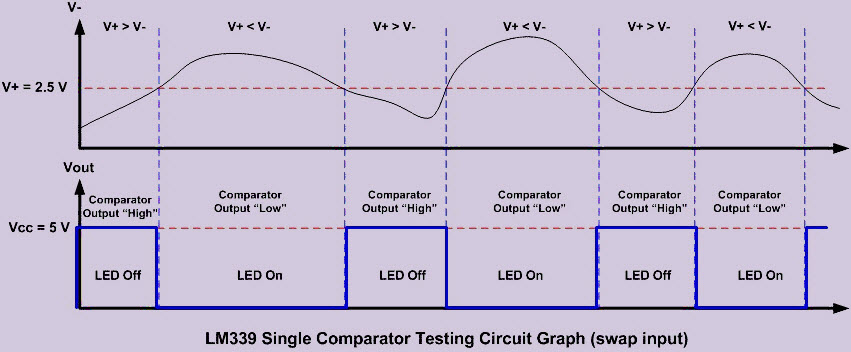# Comparator Circuit Working and Applications

Generally, in electronics, the comparator is used to compare two voltages or currents which are given at the two inputs of the comparator. That means it takes two input voltages, then compares them and gives a differential output voltage either high or low-level signal. The comparator is used to sense when an arbitrary varying input signal reaches the reference level or a defined threshold level. The comparator can be designed by using various components like diodes, transistors, op-amps. The comparators find in many electronic applications that may be used to drive logic circuits.

## Op-Amp as a Comparator

When we look closely at the comparator symbol, we will recognize it as the Op-Amp (Operational Amplifier) symbol, so what makes this comparator differ from op-amp; Op-Amp is designed to accept the analog signals and outputting the analog signal, whereas the comparator will only give output as a digital signal; although an ordinary Op-Amp could be used as the Comparators (Operational Amplifiers such as LM324, LM358, and LM741 cannot be used directly in voltage comparator circuits.

Op-Amps can often be used as voltage comparators if a diode or transistor is added to the amplifier’s output) but the real comparator is designed to have a faster switching time comparing to the multipurpose Op-Amps. Therefore, we could say that the comparator is the modified version of the Op-Amps which specially designed to give the digital output.

### Basic Comparator Circuit Working

The comparator circuit work by simply taking two analog input signals, comparing them and then produce the logical output high “1” or low “0“.

By applying the analog signal to the comparator + input called “non-inverting” and – input called “inverting“, the comparator circuit will compare this two analog signals, if the analog input on non-inverting input is greater than the analog input on inverting then the output will swing to the logical high and this will make the open collector transistor Q8 on the LM339 equivalent circuit above to turn ON. When the analog input on non-inverting is less than the analog input on inverting input, then the comparator output will swing to the logical low.

This will make Q8 transistor turn OFF. As we have seen from the LM339 equivalent circuit picture above, the LM339 uses an open collector transistor Q8 in its output, therefore we have to use the “pull-up” resistor which is connected to the Q8 collector lead with the Vcc in order to make this Q8 transistor work. According to the LM339 datasheet, the maximum current that could flow on this Q8 transistor (output sink current) is about 18 mA. The V- could be calculated as follows.

V- = R2.Vcc / (R1 + R2)

The comparator non-inverting input is connected to the 10 K potentiometer, which is also forming the voltage divider circuit where we could adjust the V+ voltage start from Vcc down to 0 volts. First, when the V+ is equal to Vcc the comparator output will swing to the logical high (Vout = Vcc) because the V+ is greater than V-.

This will switch the Q8 transistor OFF and the LED will turn OFF. When the voltage V+ drop bellow V- volts, the comparator output will swing to the logical low (Vout = GND) and this will switch the Q8 transistor ON and the LED will turn ON.By swapping the analog input; the R1 and R2 voltage divider connected to the non-inverting input (V+) and the potentiometer connected to the inverting input (V-) we will get the opposite output result.

Again, using the voltage divider principle the voltage on the non-inverting input (V+) is about V- volts, therefore if we start the inverting input voltage (V-) at Vcc volts, the V+ is lower than the V-, this will make the Q8 transistor ON the comparator output will swing to the logical low. When we adjust the V- down bellow the V+. Then the Q8 transistor OFF the comparator output will swing to the logical high because the V+ now is greater than V- and the LED will turn OFF.## Application of Comparator in Practical Electronics Circuits

### The humidity monitoring system of soil based on wireless sensor networks using Arduino

The humidity monitoring system of soil based on wireless sensor networks using the Arduino project is designed for developing an automatic irrigation system that can control the switching operation (on/off) pump motor depending upon the moisture content in the soil.

The moisture sensor senses the moisture of soil and an appropriate signal is given to the Arduino board. The comparator will compare moisture level signals with the predefined reference signal. Then it will send a signal to the microcontroller. Based on the signal received from the sensing arrangement and comparator signal, the water pump will be operated. The LCD display is used for displaying the status of soil moisture content and water pump.

### Heartbeat Sensor Circuit

HRM-2511E heartbeat sensor has 4 op-amps. The fourth Opamp is used as a voltage comparator. The analog PPG signal is fed to the positive input and the negative input is tied to a reference voltage (VR). The magnitude of VR can be set anywhere between 0 and Vcc through potentiometer P2 (shown above). Every time the PPG pulse wave exceeds the threshold voltage VR, the output of the comparator goes high. Thus, this arrangement provides an output digital pulse which is synchronized to the heartbeat. The width of the pulse is also determined by the threshold voltage VR.